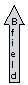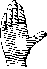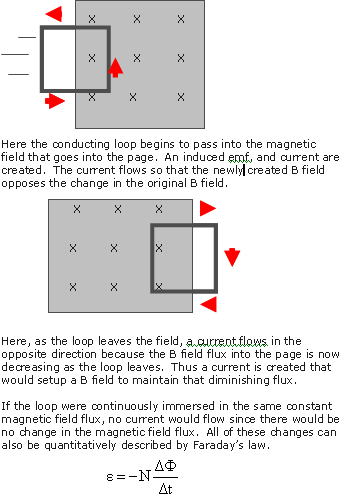HOME COURSES PREVIEW REVIEW ABOUT CONTACTTOLL-FREE INFO & ORDERING: M-F: 9am-5pm (PST): (877) RAPID-10

 Quick Search: Keywords:

 Rapid Learning Member Area:Note: If you are a legacy user of chemistry24 members, please request a new login access to the premium server with your full name and old login email via vip@rapidlearningcenter.com

 Rapid Courses Catalog : Physics in 24 Hours Chemistry in 24 Hours Biology in 24 Hours Mathematics in 24 Hours

Weekly Physics Tips:
Want to become a top gun in your class? How about study less yet score high? Sign up this Physics Survival Weekly to learn how. Designed specifically for students who are taking physics, this free newsletter will show you how to survive and excel in class! Weekly topics include:
• How to Study Physics Effectively
• How to Read Physics Textbooks Easily
• How to Solve Physics Problems Systematically
• How to Score High on Physics Exams Strategically
• How to Master Physics Rapidly
Each week, you will receive study tips on the topics above and visual tutorial or study template to enhance your physics learning. Enter your name and email below to subscribe free:

 Physics Study Lounge These study sheets are for quick review on the subjects. Refer to our rapid courses for comprehensive review.     - Basic Skills in Physics     - Basic Math for Physics     - How to Solve Physics Problems     - Newton's Laws Study Guide     - ElectroMagnetism Quick Review     - Atomic Physics At-A-Glance     - Core Concepts in Nuclear Physics     - Special Relativity Overview

 Other Related Sites
 Note: For course links to launch, disable popup blockers or hold the ctrl key while clicking the link.

ElectroMagnetism Quick Review

Key Physics Terms

• Charge:  A fundamental intrinsic property of matter that gives rise to the attractions and repulsions between electrons and protons.
• Electron: A small, light negative particle in the shell of an atom.
• Current: Electrical charge flow past a given point per unit of time.
• Magnetic Field Lines:  Lines showing the shape and exent of a magnetic field around a permanent magnet or a moving charged object.
• Magnetic Flux: A measurement of the number of magnetic field lines passing through a particular area or surface.
• Faraday’s Law: The voltage induced is directly proportionoal to the number of loops and the change in the magnetic flux.  It is inversely proportional to the time that this change occurs throughout.
• Electromotive Force, EMF:  A voltage that gives rise to a current flow.  This voltage can be induced or created by a changing magnetic field.
• Induced current:  The flow of charge in a conductor due to the changing magnetic flux near that conductor.
• Lenz’s Law: The induced emf always gives rise to a current whose magnetic flux opposed the original change in magnetic flux.  Thus, the induced current tries to maintain the level of magnetic flux.
• Generator:  A machine that produces electricity by a rotating coil of wire immersed in a stationary magnetic field.  This rotating motion could be obtained from a variety of sources.
• Right Hand Rule:  The fingers extend or curl in the direction of the magnetic field.  The outstretched thumb points in the direction of conventional current.

Key Formulas and Constants

• FB=BAcosθ
• Acircle=∏r2
• ε=-NΔF/Δt
• ε=BLv
• v=d/t

Variables Used

• I=current
• FB=magnetic flux
• ΔF=change in magnetic flux over a period of time
• A=area of surface or loop through which flux flows
• B=Magentic field strength
• θ = angle between a normal to the surface, and the B field
• ε=induced electromotive force, emf
• N= number of coils of conductor
• Δt=change in time through which B field changes
• v=velocity of a conductor moving in a B field
• L=length of conductor in B field

Typical Key Metric Units

• Current: Amperes, Amps, A
• Magnetic field: Teslas, T
• Magnetic flux: Tm2, Webers, Wb
• Area: meters squared, m2
• Length: meters, m
• EMF: volts, V
• Angle: degrees
• Distance or radius: meters, m
• Velocity: meters per second, m/s
• Time: seconds, s

Electromagnetism Problem Solving Tips

• These tips will make it easier to solve any physics problems.
• Thoroughly read the entire problem.
• Draw a diagram if needed.
• Identify all given information.
• Identify the quantity to be found.
• Select appropriate formula(s) that incorporate what you know and what you want to find.
• Be sure to consider the direction of the B field and flux when deciding upon a current direction.
• Convert units if needed.
• Do any mathematical calculations carefully.

Right Hand Rule DiagramsExamples of Lenz’s Law